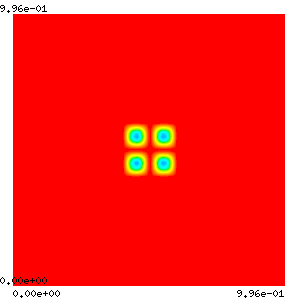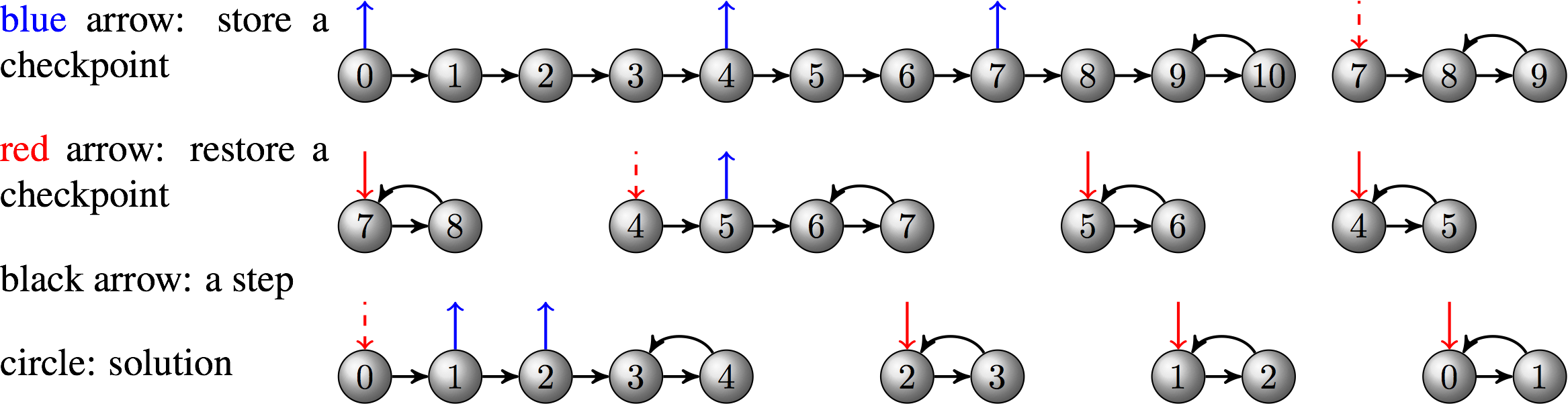# Using adjoint for PDE-constrained optimization

## At a Glance

 Questions Objectives Key Points How can gradients be computed for simulations? Know PETSc/TAO’s capability for adjoint and optimization Adjoint enables dynamic constrained optimization How difficult is it to use the adjoint method? Understand ingredients needed for adjoint calculation Jacobian is imperative Understand the concern of checkpointing Performance may depend on checkpointing at large scale

Note: To begin this lesson…

cd HandsOnLessons/adjoint


## An Inverse Initial Value Problem

This code demonstrates how to solve an inverse initial value problem for a system of time-dependent PDEs on a 2D rectangular grid. The goal is to determine an optimal initial condition that can minimizes the difference between the simulated result and the reference solution. We will use this example to illustrate the performance considerations for realistic large-scale applications. In particular, we will show how to play with checkpointing and how to profile/tune the performance.

### Compile the code

The example ex5opt_ic.c can be compiled with

make ex5opt_ic


To clean the example, do

make clean


ATPESC participants do not need to compile code because binaries are available in the ATPESC project folder on Cooley.

### Command line options

You can determine the command line options available for this particular example by doing

./ex5opt_ic -help


and show the options related to TAO only by doing

./ex5opt_ic -help | grep tao


### Problem being solved

The underlying PDE models reaction and diffusion of two chemical species that can produce a variety of patterns. It is widely used to describe pattern-formation phenomena in biological, chemical and physical systems. The concentrations of the two species are calculated according to the equation $\frac{d\mathbf{u}}{dt} = D_1 \nabla^2 \mathbf{u} - \mathbf{u} \mathbf{v}^2 + \gamma(1 -\mathbf{u}) \\ \frac{d\mathbf{v}}{dt} = D_2 \nabla^2 \mathbf{v} + \mathbf{u} \mathbf{v}^2 - (\gamma + \kappa)\mathbf{v}$

The spatial pattern for the time interval [0,200] seconds is shown in the following figure.

Figure 1Given the pattern (observation) at the final time of the simulation, we want to determine the initial pattern that can minimize the difference between the simulated result and the observation. $\text{minimize}_{X_0} \| X - X^{ref}\|$

### Run 1: Monitor solution graphically

mpiexec -n 4 ./ex5opt_ic -forwardonly -ts_type rk -ts_rk_type 3 -ts_max_steps 20 -ts_monitor -ts_monitor_draw_solution

• -forwardonly perform the forward simulation without doing optimization
• -ts_type rk -ts_rk_type 3 changes the time stepping algorithm to a 3rd-order Runge-Kutta method
• -ts_monitor_draw_solution monitors the progress for the solution at each time step
• Add -draw_pause -2 if you want to pause at the end of simulation to see the plot

### Run 2: Optimal checkpointing schedule

By default, the checkpoints are stored in binary files on disk. Of course, this may not be a good choice for large-scale applications running on high-performance machines where I/O cost is significant. We can make the solver use RAM for checkpointing and specify the maximum allowable checkpoints so that an optimal adjoint checkpointing schedule that minimizes the number of recomputations will be generated.

mpiexec -n 4 ./ex5opt_ic -ts_type rk -ts_rk_type 3 -ts_adapt_type none \
-ts_trajectory_type memory -ts_trajectory_max_cps_ram 3 \
-ts_trajectory_monitor -ts_trajectory_view -tao_max_it 1

• -tao_max_it 1 forces the optimization to stop after one iteration so that we can focus on the output related to the adjoint checkpointing. The output corresponds to the schedule depicted by the following diagram:#### Questions

Looking at the output, we will find that the new schedule uses both RAM and disk for checkpointing and takes two less recomputations.

### Run 3: Monitor the optimization progress

mpiexec -n 4 ./ex5opt_ic -ts_type rk -ts_rk_type 3 -ts_adapt_type none -ts_max_steps 5 -ts_trajectory_type memory -ts_trajectory_solution_only 0 -tao_monitor -tao_view
0 TAO,  Function value: 8.48202,  Residual: 7.02449
1 TAO,  Function value: 1.72589,  Residual: 2.46827
2 TAO,  Function value: 0.556599,  Residual: 1.75419
3 TAO,  Function value: 0.181113,  Residual: 0.698038
4 TAO,  Function value: 0.0919628,  Residual: 0.390951
5 TAO,  Function value: 0.0330733,  Residual: 0.276822
6 TAO,  Function value: 0.0137442,  Residual: 0.205942
7 TAO,  Function value: 0.0032463,  Residual: 0.0925536
8 TAO,  Function value: 0.000876358,  Residual: 0.0469696
9 TAO,  Function value: 0.000172022,  Residual: 0.0205954
10 TAO,  Function value: 2.32065e-05,  Residual: 0.00808249
11 TAO,  Function value: 1.00524e-05,  Residual: 0.00695292
12 TAO,  Function value: 2.19094e-06,  Residual: 0.00192488
13 TAO,  Function value: 9.37506e-07,  Residual: 0.00115926
14 TAO,  Function value: 2.79883e-07,  Residual: 0.000907609
15 TAO,  Function value: 7.22e-08,  Residual: 0.000346602
16 TAO,  Function value: 3.51499e-08,  Residual: 0.000184429
17 TAO,  Function value: 1.48741e-08,  Residual: 0.000117587
18 TAO,  Function value: 7.83221e-09,  Residual: 9.19265e-05
19 TAO,  Function value: 2.14226e-09,  Residual: 4.54118e-05
20 TAO,  Function value: 8.99022e-10,  Residual: 2.91421e-05
21 TAO,  Function value: 3.28239e-10,  Residual: 2.06652e-05
22 TAO,  Function value: 1.85795e-10,  Residual: 2.71364e-05
23 TAO,  Function value: 1.14498e-10,  Residual: 2.32933e-05
24 TAO,  Function value: 8.12369e-11,  Residual: 2.22391e-05
25 TAO,  Function value: 1.87747e-11,  Residual: 7.85018e-06
26 TAO,  Function value: 7.29614e-12,  Residual: 5.02828e-06
27 TAO,  Function value: 1.72522e-12,  Residual: 1.07136e-06
28 TAO,  Function value: 1.0259e-12,  Residual: 7.63383e-07
29 TAO,  Function value: 1.72785e-13,  Residual: 3.91911e-07
30 TAO,  Function value: 1.0509e-13,  Residual: 2.75909e-07
31 TAO,  Function value: 4.02491e-14,  Residual: 1.7222e-07
32 TAO,  Function value: 1.5385e-14,  Residual: 1.21345e-07
33 TAO,  Function value: 3.20145e-15,  Residual: 7.81137e-08
34 TAO,  Function value: 1.66953e-15,  Residual: 5.25802e-08
35 TAO,  Function value: 3.39432e-16,  Residual: 3.16327e-08
36 TAO,  Function value: 9.68078e-17,  Residual: 1.15783e-08
37 TAO,  Function value: 8.23443e-17,  Residual: 1.68247e-08
38 TAO,  Function value: 1.53356e-17,  Residual: 7.63537e-09
Tao Object: 4 MPI processes
type: blmvm
Mat Object: (tao_blmvm_) 4 MPI processes
type: lmvmbfgs
rows=8192, cols=8192
Scale type: diagonal
Scale history: 1
Scale params: alpha=1., beta=0.5, rho=1.
Convex factors: phi=0., theta=0.125
Max. storage: 5
Used storage: 5
Number of rejects: 0
Number of resets: 1
TaoLineSearch Object: 4 MPI processes
type: more-thuente
maximum function evaluations=30
tolerances: ftol=0.0001, rtol=1e-10, gtol=0.9
total number of function evaluations=0
using variable bounds
Termination reason: 1
Active Set subset type: subvec
convergence tolerances: gatol=1e-08,   steptol=0.,   gttol=0.
Objective value=1.53356e-17
total number of iterations=38,                          (max: 2000)
total number of function/gradient evaluations=41,      (max: 4000)
Solution converged:    ||g(X)|| <= gatol

• -tao_draw_solution can visualize the solution at each optimization iteration

#### Questions

Essential functions we have provided are FormFunctionGradient for TAO, TSIFunction and TSIJacobian for TS, RHSJacobianP for TSAdjoint. Because of the integral in the objective function, extra functions including CostIntegrand, DRDYFunction and DRDPFunction are given to TSAdjoint.

### Run 4: Implicit time integration method

Now we switch to an implicit method (Crank-Nicolson) using fixed stepsize, which is the default setting in the code. At each time step, a nonlinear system is solved by the PETSc nonlinear solver SNES.

mpiexec -n 12 ./ex5opt_ic -ts_max_steps 5 -log_view -tao_monitor

• -snes_monitor can show the progress of SNES
• -ts_monitor can show the progress of TS
• -log_view prints a summary of the logging

A snippet of the summary:

...
Phase summary info:
Count: number of times phase was executed
Time and Flop: Max - maximum over all processors
Ratio - ratio of maximum to minimum over all processors
Mess: number of messages sent
Avg. len: average message length (bytes)
Reduct: number of global reductions
Global: entire computation
Stage: stages of a computation. Set stages with PetscLogStagePush() and PetscLogStagePop().
%T - percent time in this phase         %F - percent flop in this phase
%M - percent messages in this phase     %L - percent message lengths in this phase
%R - percent reductions in this phase
Total Mflop/s: 10e-6 * (sum of flop over all processors)/(max time over all processors)
------------------------------------------------------------------------------------------------------------------------
Event                Count      Time (sec)     Flop                             --- Global ---  --- Stage ---   Total
Max Ratio  Max     Ratio   Max  Ratio  Mess   Avg len Reduct  %T %F %M %L %R  %T %F %M %L %R Mflop/s
------------------------------------------------------------------------------------------------------------------------

--- Event Stage 0: Main Stage

VecDot              1130 1.0 3.9795e-01 1.4 1.59e+06 1.0 0.0e+00 0.0e+00 1.1e+03  4  1  0  0  8   4  1  0  0  8    46
VecMDot             1919 1.0 5.9272e-01 2.0 5.40e+06 1.0 0.0e+00 0.0e+00 1.9e+03  5  4  0  0 14   5  4  0  0 14   106
VecNorm             3678 1.0 8.1644e-01 1.3 5.18e+06 1.0 0.0e+00 0.0e+00 3.7e+03  9  4  0  0 27   9  4  0  0 27    74
...


#### Questions

Of course answer may vary depending on the settings such as number of procs, problem size, and solver options. Typically most of the time should be spent on VecMDot or MatMult

1. Use memory instead of disk for checkpointing(-ts_trajectory_type memory -ts_trajectory_solution_only 0); 2. Tune the time stepping solver, nonlinear solver, linear solver, preconditioner and so forth.

### Run 5: Scale up the problem

We use explicit Runge-Kutta methods for time integration, and increase the grid resolution to 512 X 512.

mpiexec -n 12 ./ex5opt_ic -ts_type rk -ts_rk_type 3 -ts_adapt_type none -ts_max_steps 5 -ts_trajectory_type memory -ts_trajectory_solution_only 0 -tao_monitor -da_grid_x 512 -da_grid_y 512


#### Questions

No. The PDE solution blows up. As we decrease the grid spacing, the stepsize should be reduced according to CFL condition. For example, adding -ts_dt 0.1 should work.

### Further information

Because this example uses DMDA, Jacobian can be efficiently approximated using finite difference with coloring. You can use the option -snes_fd_color to enable this feature.

## Out-Brief

We have used PETSc to demonstrate the adjoint capability as an enabling technology for dynamic-constrained optimization. In particular, we investigated an inverse initial value porblem built on a time-dependent reaction-diffusion PDE.

We have shown the basic usage of the adjoint solver as well as functionalities that can facilitate rapid development, diagnosis and performance profiling.